# RD Sharma Solutions Class 12 The Plane Exercise 29.2

RD Sharma Solutions for Class 12 Maths Chapter 29 The Plane Exercise 29.2, is provided here. The solutions are created by subject-matter experts having vast experience in the academic field, which helps students attain good marks in exams. Practising these RD Sharma Class 12 Solutions regularly will help students attain good scores in the board exams. Students can easily download the pdf consisting of this chapter solutions, which are available in the links provided below and can use it for future reference as well.

## Download PDF of RD Sharma Solutions For Class 12 Maths Chapter 29 Exercise 2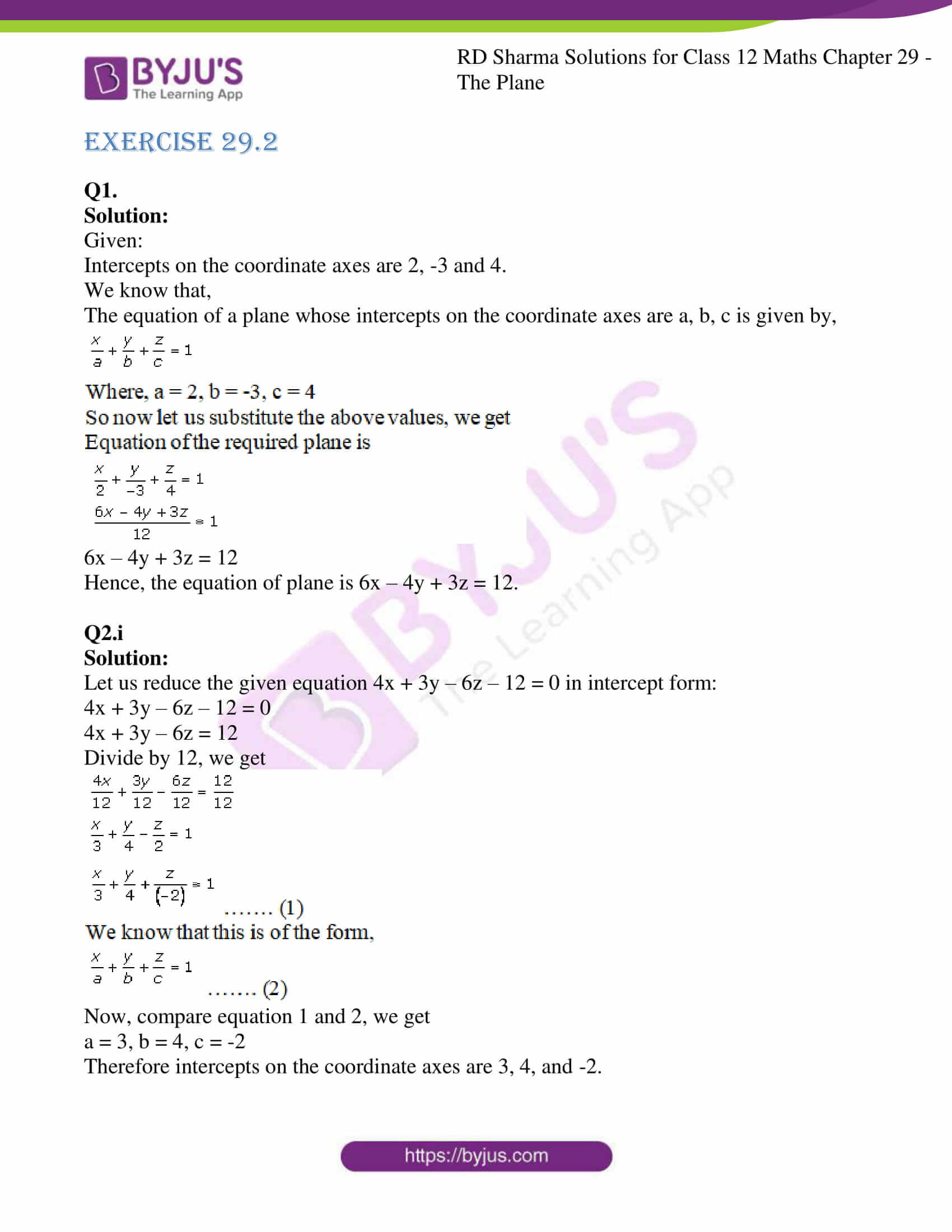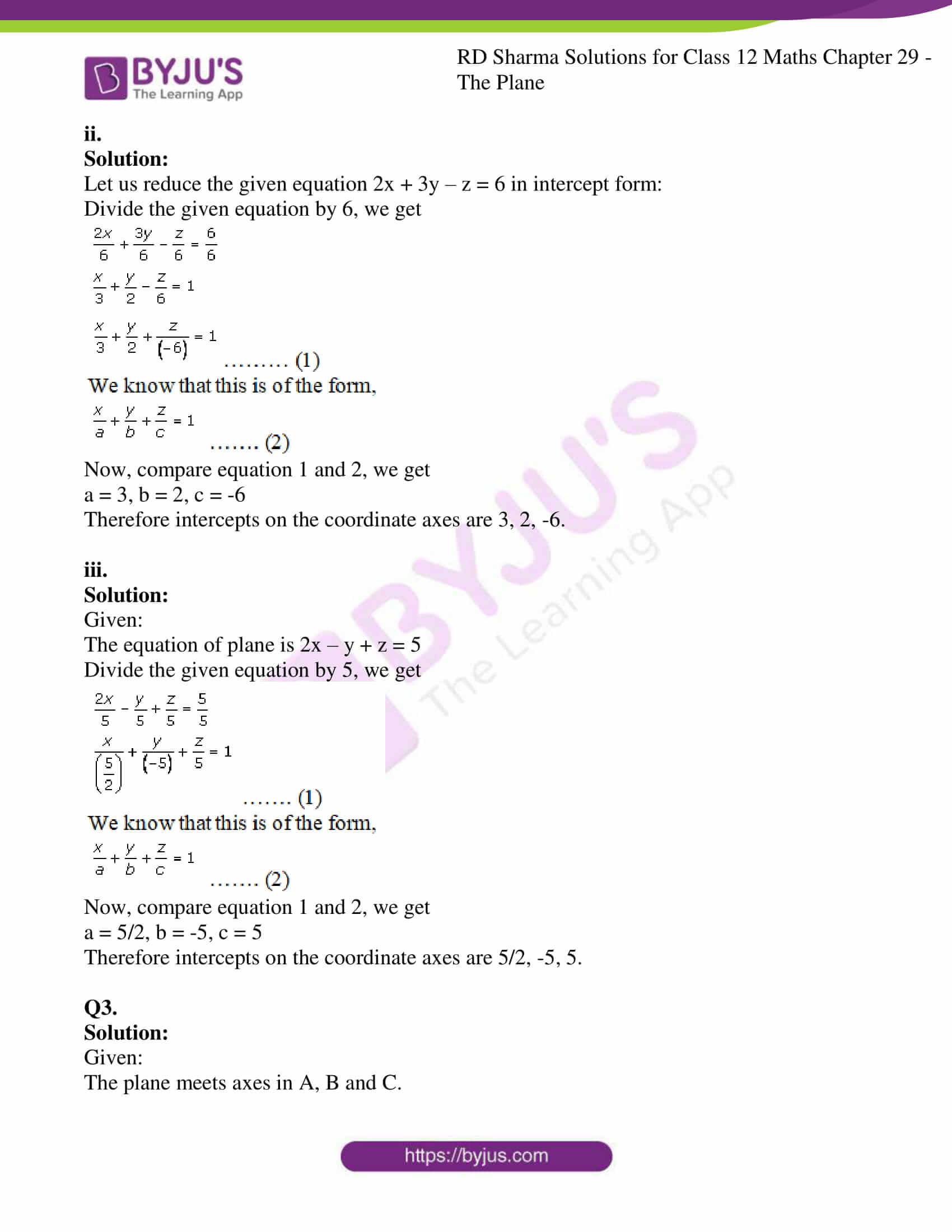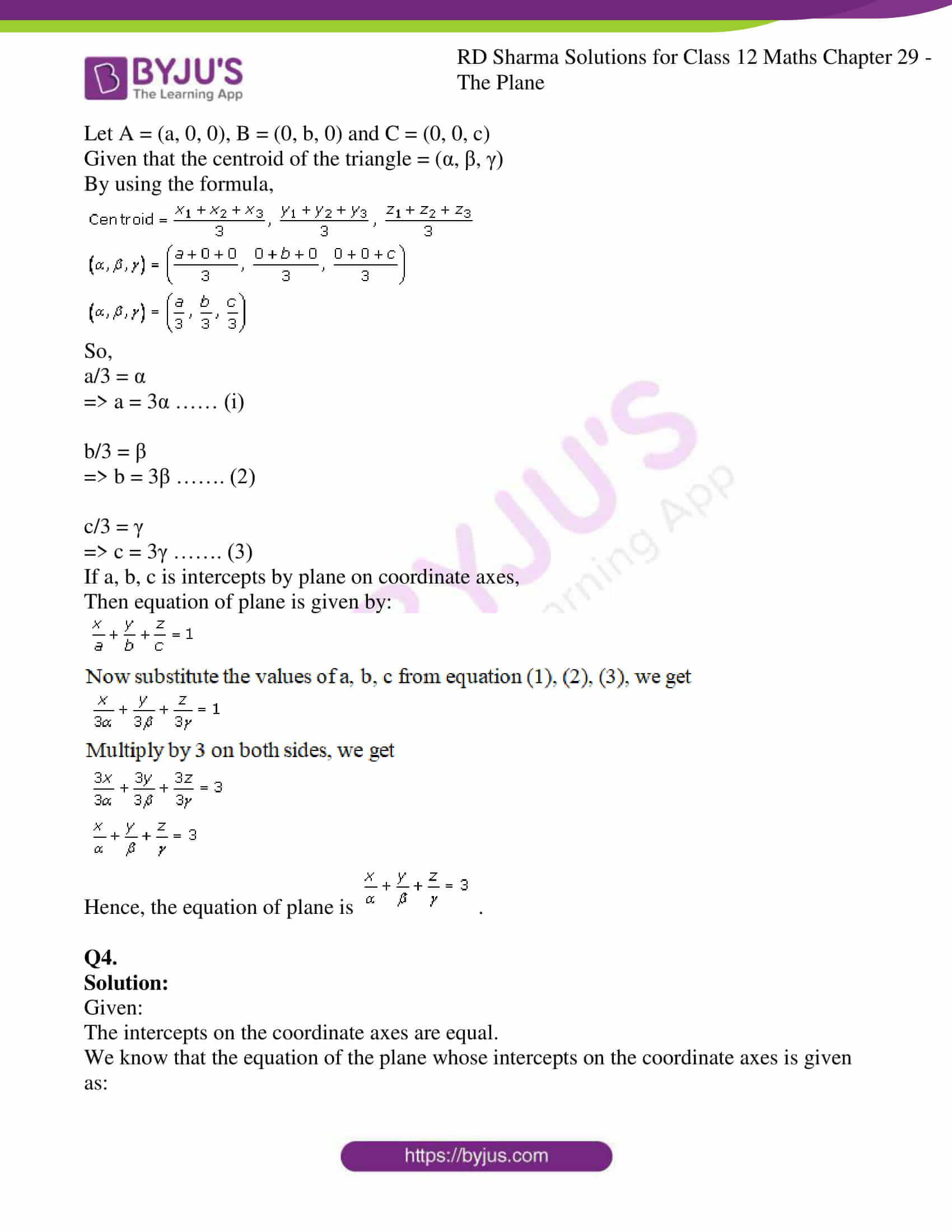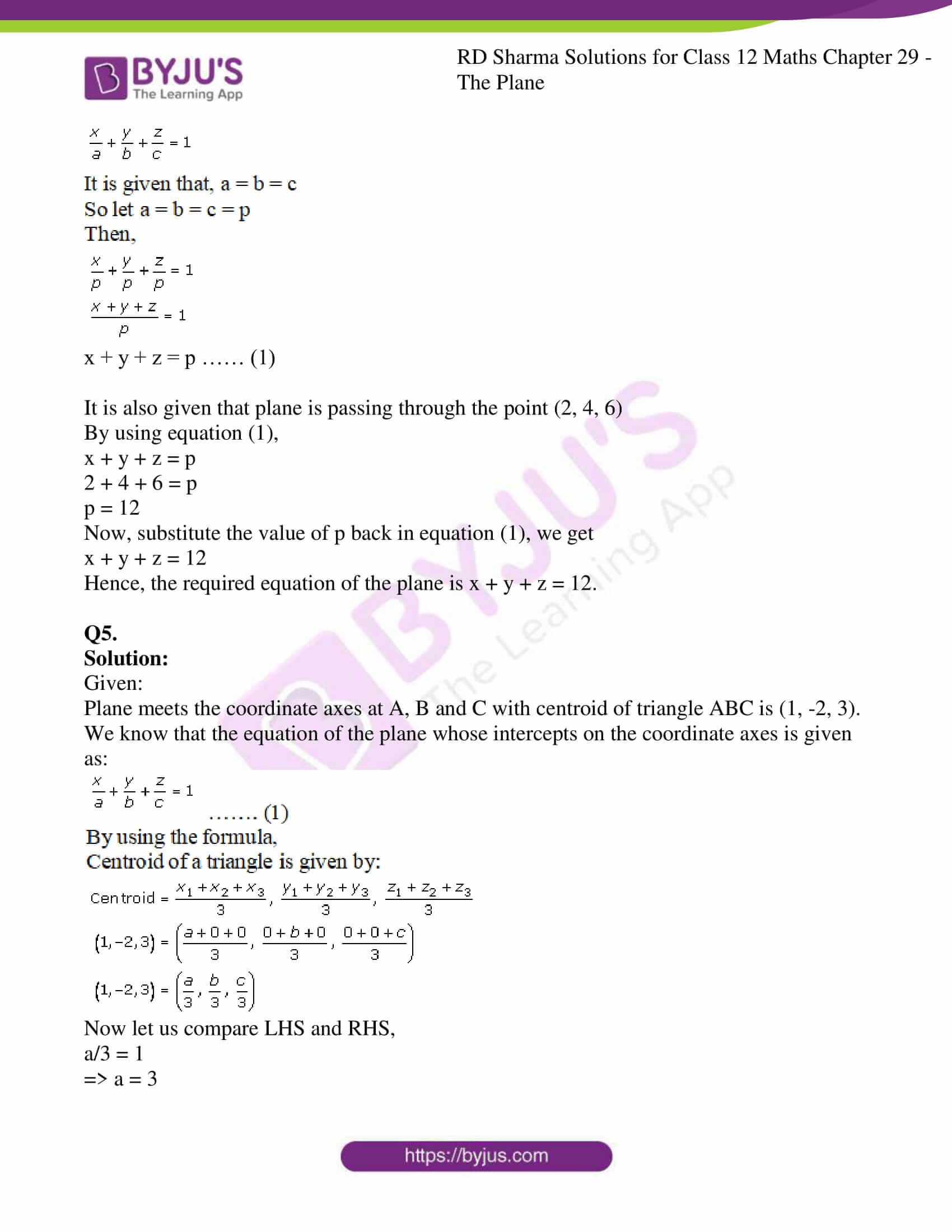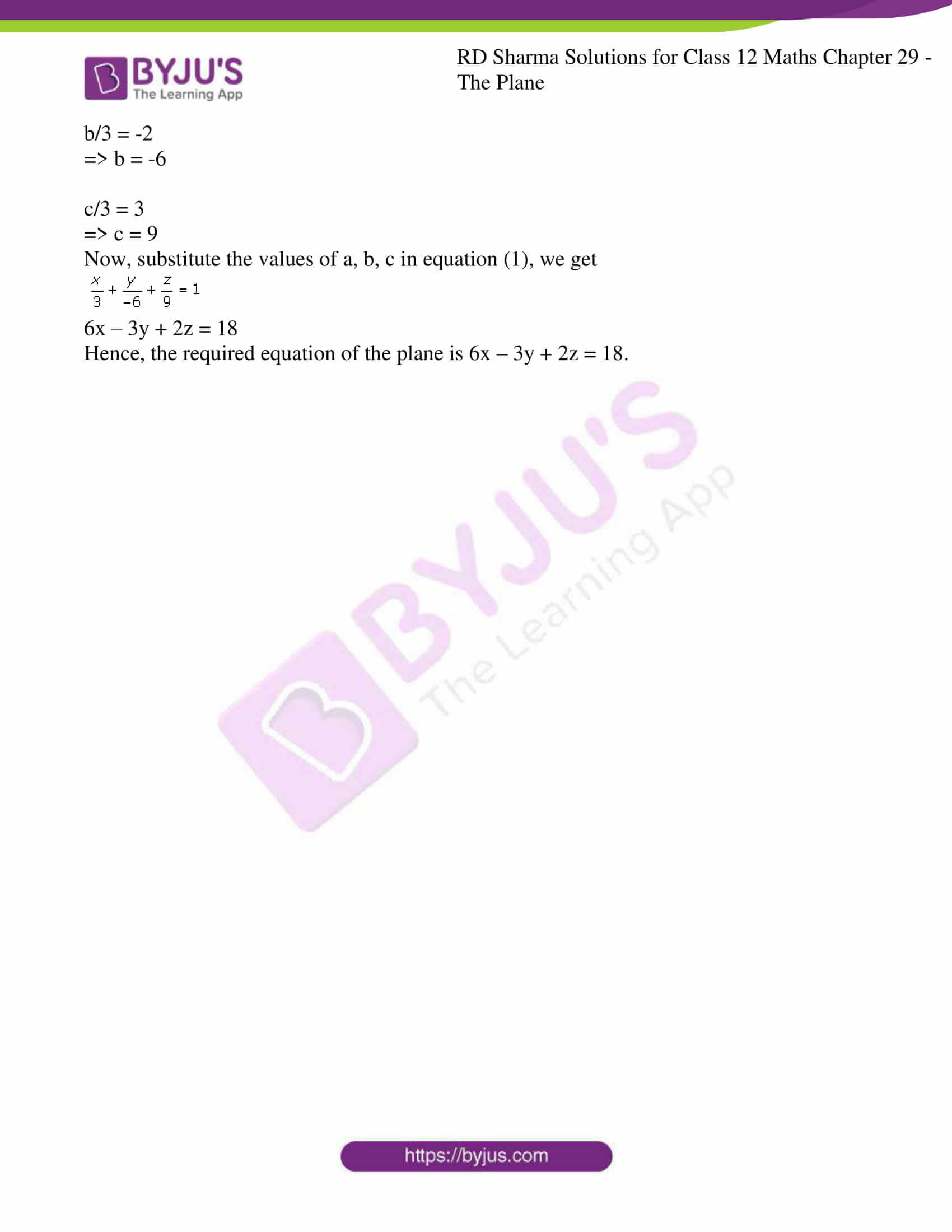### Access RD Sharma Solutions For Class 12 Maths Chapter 29 Exercise 2

Q1.

Solution:

Given:

Intercepts on the coordinate axes are 2, -3 and 4.

We know that,

The equation of a plane whose intercepts on the coordinate axes are a, b, c is given by,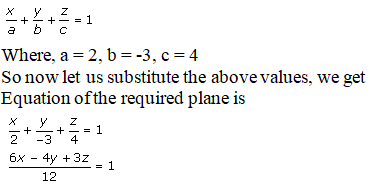6x – 4y + 3z = 12

Hence, the equation of plane is 6x – 4y + 3z = 12.

Q2.i

Solution:

Let us reduce the given equation 4x + 3y – 6z – 12 = 0 in intercept form:

4x + 3y – 6z – 12 = 0

4x + 3y – 6z = 12

Divide by 12, we get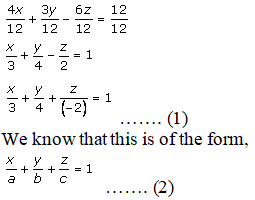Now, compare equation 1 and 2, we get

a = 3, b = 4, c = -2

Therefore intercepts on the coordinate axes are 3, 4, and -2.

ii.

Solution:

Let us reduce the given equation 2x + 3y – z = 6 in intercept form:

Divide the given equation by 6, we get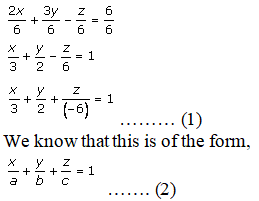Now, compare equation 1 and 2, we get

a = 3, b = 2, c = -6

Therefore intercepts on the coordinate axes are 3, 2, -6.

iii.

Solution:

Given:

The equation of plane is 2x – y + z = 5

Divide the given equation by 5, we get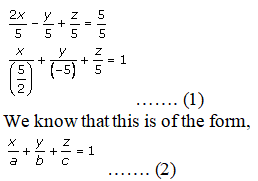Now, compare equation 1 and 2, we get

a = 5/2, b = -5, c = 5

Therefore intercepts on the coordinate axes are 5/2, -5, 5.

Q3.

Solution:

Given:

The plane meets axes in A, B and C.

Let A = (a, 0, 0), B = (0, b, 0) and C = (0, 0, c)

Given that the centroid of the triangle = (α, β, γ)

By using the formula,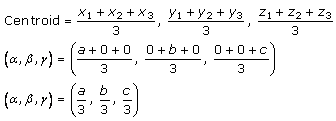So,

a/3 = α

=> a = 3α …… (i)

b/3 = β

=> b = 3β ……. (2)

c/3 = γ

=> c = 3γ ……. (3)

If a, b, c is intercepts by plane on coordinate axes,

Then equation of plane is given by: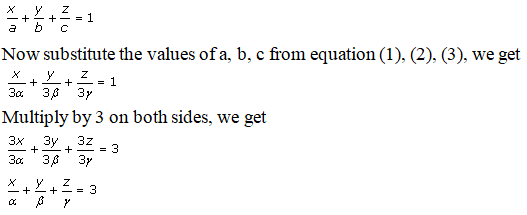Hence, the equation of plane is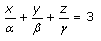.

Q4.

Solution:

Given:

The intercepts on the coordinate axes are equal.

We know that the equation of the plane whose intercepts on the coordinate axes is given as: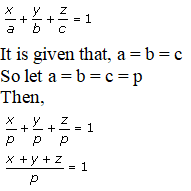x + y + z = p …… (1)

It is also given that plane is passing through the point (2, 4, 6)

By using equation (1),

x + y + z = p

2 + 4 + 6 = p

p = 12

Now, substitute the value of p back in equation (1), we get

x + y + z = 12

Hence, the required equation of the plane is x + y + z = 12.

Q5.

Solution:

Given:

Plane meets the coordinate axes at A, B and C with centroid of triangle ABC is (1, -2, 3).

We know that the equation of the plane whose intercepts on the coordinate axes is given as: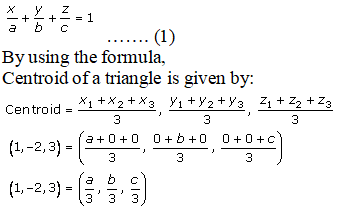Now let us compare LHS and RHS,

a/3 = 1

=> a = 3

b/3 = -2

=> b = -6

c/3 = 3

=> c = 9

Now, substitute the values of a, b, c in equation (1), we get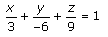6x – 3y + 2z = 18

Hence, the required equation of the plane is 6x – 3y + 2z = 18.